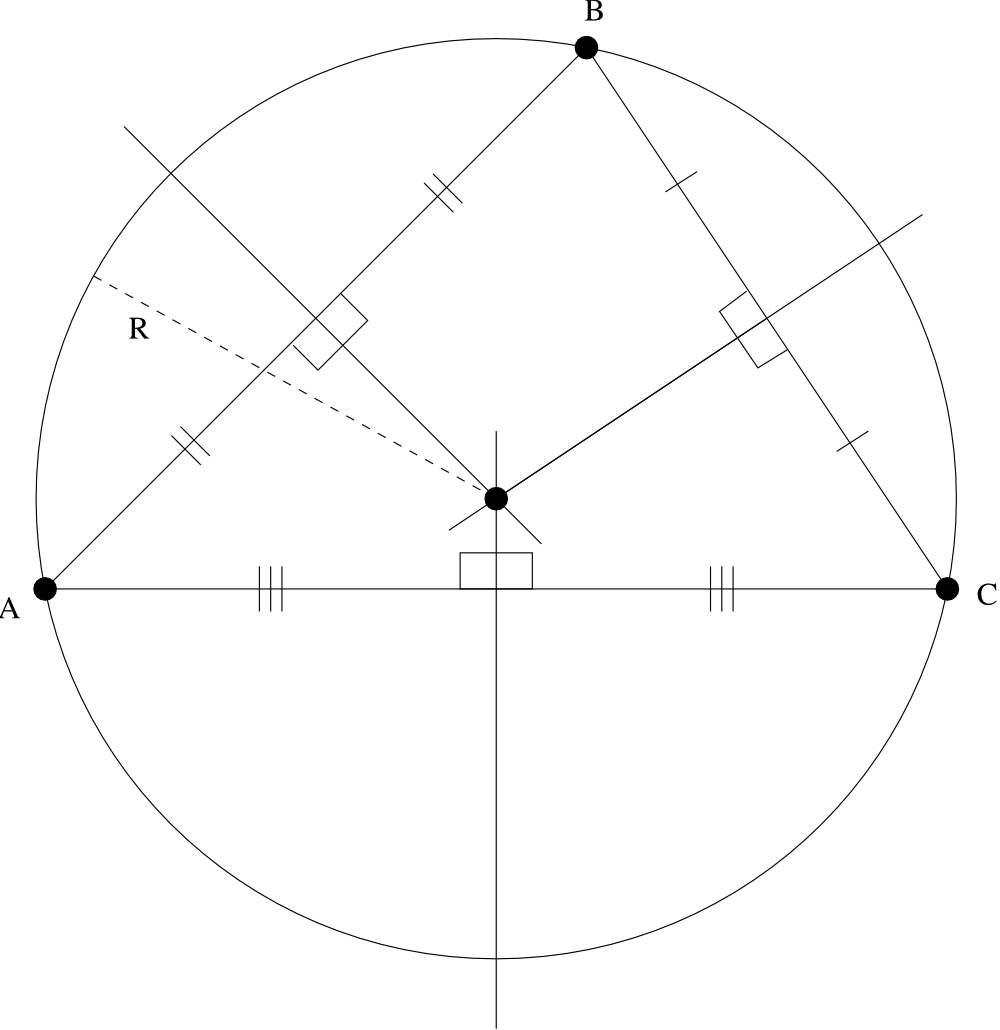시간 제한메모리 제한제출정답맞힌 사람정답 비율
1 초 128 MB3114836.364%

## 문제

Your team is to write a program that, given the Cartesian coordinates of three points on a plane, will find the equation of the circle through them all. The three points will not be on a straight line.

The solution is to be printed as an equation of the form

$(x - h)^{2} + (y - k)^{2} = r^{2}$

and an equation of the form

$x^{2} + y^{2} + cx + dy - e = 0$

## 입력

Each line of input to your program will contain the $$x$$ and $$y$$ coordinates of three points, in the order $$A_{x}$$, $$A_{y}$$, $$B_{x}$$, $$B_{y}$$, $$C_{x}$$, $$C_{y}$$.  These coordinates will be real numbers separated from each other by one or more spaces.

## 출력

Your program must print the required equations on two lines using the format given in the sample below. Your computed values for $$h$$, $$k$$, $$r$$, $$c$$, $$d$$, and $$e$$ in Equations 1 and 2 above are to be printed with three digits after the decimal point. Plus and minus signs in the equations should be changed as needed to avoid multiple signs before a number. Plus, minus, and equal signs must be separated from the adjacent characters by a single space on each side. No other spaces are to appear in the equations. Print a single blank line after each equation pair.

## 예제 입력 1

7.0 -5.0 -1.0 1.0 0.0 -6.0
1.0 7.0 8.0 6.0 7.0 -2.0


## 예제 출력 1

(x - 3.000)^2 + (y + 2.000)^2 = 5.000^2
x^2 + y^2 - 6.000x + 4.000y - 12.000 = 0

(x - 3.921)^2 + (y - 2.447)^2 = 5.409^2
x^2 + y^2 - 7.842x - 4.895y - 7.895 = 0


## 힌트